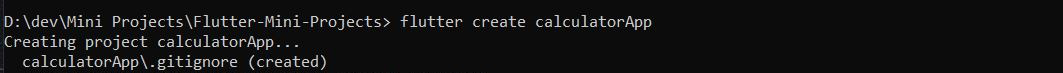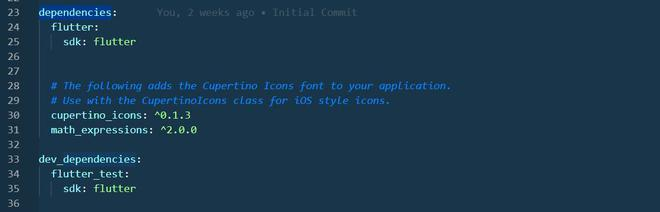# Simple Calculator App using Flutter

• Difficulty Level : Easy
• Last Updated : 04 Oct, 2021

Flutter SDK is an open-source software development kit for building beautiful UI which is natively compiled. In this article we will build a Simple Calculator App that can perform basic arithmetic operations like addition, subtraction, multiplication or division depending upon the user input. Making this app will give you a good revision of flutter and dart basics. Concepts covered are:

• Showing Widgets on the screen.
• Gridview.builder
• Function writing
• If and else in dart

Follow the below steps to implement the simple calculator. Let’s get started.

Want a more fast-paced & competitive environment to learn the fundamentals of Android?
Click here to head to a guide uniquely curated by our experts with the aim to make you industry ready in no time!

#### Step 1: Creating a Flutter App

Open the Terminal /Command-prompt. Change Directory to your choice and run flutter create calculatorApp. Open the calculatorApp in VS Code or Android Studio.#### Step 2: Coding Calculator App

In the Lib folder, there is a main.dart file already present. And now in the same folder create a new file named buttons.dart. Starting with main.dart file. Create MyApp class and make it StatelessWidget. Add an array of buttons to be displayed. Set the background-color, text-color, functionality onTapped to the buttons. Write a function to calculate the Answers

## Dart

 `import ``'package:flutter/material.dart'``;``import ``'buttons.dart'``;``import ``'package:math_expressions/math_expressions.dart'``;` `void` `main() {``  ``runApp(MyApp());``}` `class` `MyApp extends StatelessWidget {``  ``@override``  ``Widget build(BuildContext context) {``    ``return` `MaterialApp(``      ``debugShowCheckedModeBanner: ``false``,``      ``home: HomePage(),``    ``); ``// MaterialApp``  ``}``}` `class` `HomePage extends StatefulWidget {``  ``@override``  ``_HomePageState createState() => _HomePageState();``}` `class` `_HomePageState extends State {``  ``var userInput = ``''``;``  ``var answer = ``''``;` `  ``// Array of button``  ``final List buttons = [``    ``'C'``,``    ``'+/-'``,``    ``'%'``,``    ``'DEL'``,``    ``'7'``,``    ``'8'``,``    ``'9'``,``    ``'/'``,``    ``'4'``,``    ``'5'``,``    ``'6'``,``    ``'x'``,``    ``'1'``,``    ``'2'``,``    ``'3'``,``    ``'-'``,``    ``'0'``,``    ``'.'``,``    ``'='``,``    ``'+'``,``  ``];` `  ``@override``  ``Widget build(BuildContext context) {``    ``return` `Scaffold(``      ``appBar: ``new` `AppBar(``        ``title: ``new` `Text(``"Calculator"``),``      ``), ``//AppBar``      ``backgroundColor: Colors.white38,``      ``body: Column(``        ``children: [``          ``Expanded(``            ``child: Container(``              ``child: Column(``                  ``mainAxisAlignment: MainAxisAlignment.spaceEvenly,``                  ``children: [``                    ``Container(``                      ``padding: EdgeInsets.all(20),``                      ``alignment: Alignment.centerRight,``                      ``child: Text(``                        ``userInput,``                        ``style: TextStyle(fontSize: 18, color: Colors.white),``                      ``),``                    ``),``                    ``Container(``                      ``padding: EdgeInsets.all(15),``                      ``alignment: Alignment.centerRight,``                      ``child: Text(``                        ``answer,``                        ``style: TextStyle(``                            ``fontSize: 30,``                            ``color: Colors.white,``                            ``fontWeight: FontWeight.bold),``                      ``),``                    ``)``                  ``]),``            ``),``          ``),``          ``Expanded(``            ``flex: 3,``            ``child: Container(``              ``child: GridView.builder(``                  ``itemCount: buttons.length,``                  ``gridDelegate: SliverGridDelegateWithFixedCrossAxisCount(``                      ``crossAxisCount: 4),``                  ``itemBuilder: (BuildContext context, ``int` `index) {``                    ``// Clear Button``                    ``if` `(index == 0) {``                      ``return` `MyButton(``                        ``buttontapped: () {``                          ``setState(() {``                            ``userInput = ``''``;``                            ``answer = ``'0'``;``                          ``});``                        ``},``                        ``buttonText: buttons[index],``                        ``color: Colors.blue,``                        ``textColor: Colors.black,``                      ``);``                    ``}` `                    ``// +/- button``                    ``else` `if` `(index == 1) {``                      ``return` `MyButton(``                        ``buttonText: buttons[index],``                        ``color: Colors.blue,``                        ``textColor: Colors.black,``                      ``);``                    ``}``                    ``// % Button``                    ``else` `if` `(index == 2) {``                      ``return` `MyButton(``                        ``buttontapped: () {``                          ``setState(() {``                            ``userInput += buttons[index];``                          ``});``                        ``},``                        ``buttonText: buttons[index],``                        ``color: Colors.blue,``                        ``textColor: Colors.black,``                      ``);``                    ``}``                    ``// Delete Button``                    ``else` `if` `(index == 3) {``                      ``return` `MyButton(``                        ``buttontapped: () {``                          ``setState(() {``                            ``userInput =``                                ``userInput.substring(0, userInput.length - 1);``                          ``});``                        ``},``                        ``buttonText: buttons[index],``                        ``color: Colors.blue,``                        ``textColor: Colors.black,``                      ``);``                    ``}``                    ``// Equal_to Button``                    ``else` `if` `(index == 18) {``                      ``return` `MyButton(``                        ``buttontapped: () {``                          ``setState(() {``                            ``equalPressed();``                          ``});``                        ``},``                        ``buttonText: buttons[index],``                        ``color: Colors.orange,``                        ``textColor: Colors.white,``                      ``);``                    ``}` `                    ``//  other buttons``                    ``else` `{``                      ``return` `MyButton(``                        ``buttontapped: () {``                          ``setState(() {``                            ``userInput += buttons[index];``                          ``});``                        ``},``                        ``buttonText: buttons[index],``                        ``color: isOperator(buttons[index])``                            ``? Colors.blueAccent``                            ``: Colors.white,``                        ``textColor: isOperator(buttons[index])``                            ``? Colors.white``                            ``: Colors.black,``                      ``);``                    ``}``                  ``}), ``// GridView.builder``            ``),``          ``),``        ``],``      ``),``    ``);``  ``}` `  ``bool` `isOperator(String x) {``    ``if` `(x == ``'/'` `|| x == ``'x'` `|| x == ``'-'` `|| x == ``'+'` `|| x == ``'='``) {``      ``return` `true``;``    ``}``    ``return` `false``;``  ``}` `// function to calculate the input operation``  ``void` `equalPressed() {``    ``String finaluserinput = userInput;``    ``finaluserinput = userInput.replaceAll(``'x'``, ``'*'``);` `    ``Parser p = Parser();``    ``Expression ``exp` `= p.parse(finaluserinput);``    ``ContextModel cm = ContextModel();``    ``double` `eval = ``exp``.evaluate(EvaluationType.REAL, cm);``    ``answer = eval.toString();``  ``}``}`

In Flutter main.dart file is the entry point from which the code starts to executing. In the main.dart file firstly material design package has been imported in addition to math_expressions and buttons.dart file. Then a function runApp has been created with parameter as MyApp. After the declaration of class MyApp which is a stateless widget, the state of class MyApp has been laid out.

#### Step 3: Building the buttons.dart

In buttons.dart which is already imported in main.dart file we are declaring variables that will be used throughout the program using a constructor. The color, text color, button text, and the functionality of the button on tapped will be implemented in main.dart file

## Dart

 `import ``'package:flutter/material.dart'``;` `// creating Stateless Widget for buttons``class` `MyButton extends StatelessWidget {``  ` `  ``// declaring variables``  ``final color;``  ``final textColor;``  ``final String buttonText;``  ``final buttontapped;` `  ``//Constructor``  ``MyButton({``this``.color, ``this``.textColor, ``this``.buttonText, ``this``.buttontapped});` `  ``@override``  ``Widget build(BuildContext context) {``    ``return` `GestureDetector(``      ``onTap: buttontapped,``      ``child: Padding(``        ``padding: ``const` `EdgeInsets.all(0.2),``        ``child: ClipRRect(``          ``// borderRadius: BorderRadius.circular(25),``          ``child: Container(``            ``color: color,``            ``child: Center(``              ``child: Text(``                ``buttonText,``                ``style: TextStyle(``                  ``color: textColor,``                  ``fontSize: 25,``                  ``fontWeight: FontWeight.bold,``                ``),``              ``),``            ``),``          ``),``        ``),``      ``),``    ``);``  ``}``}`

#### Step 4: Adding dependencies in pubspec.yaml file

To make the process easier we are using math_expressions: ^2.0.0  package which is imported in main.dart file to handle all the calculations and run time error exceptions.